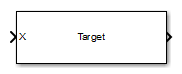## Library

Environment and Target

`phasedenvlib`

•## Description

The Radar Target block models a radar target that reflects the signal according to the specified radar cross section (RCS). The block supports all four Swerling models.

## Parameters

Source of mean radar cross section

Specify whether the target’s mean radar cross-section (RCS) value comes from the Mean radar cross section parameter of this block or from an input port. Values of this parameter are

 `Property` The Mean radar cross section parameter for this block specifies the mean RCS value. `Input port` Choosing this value creates the `RCS` input port to specify the mean radar cross-section.

Specify the mean value of the target's radar cross section, in square meters, as a nonnegative scalar. This parameter appears only when the Source of mean radar cross section parameter is set to `Property`.

Fluctuation model

Specify the statistical model of the target as one of `Nonfluctuating`, `Swerling1`, `Swerling2`, `Swerling3`, or `Swerling4`. Setting this parameter to a value other than `Nonfluctuating`, allows setting cross-sections parameters via an input port, `Update`.

Signal Propagation speed (m/s)

Specify the propagation speed of the signal, in meters per second, as a positive scalar. You can use the function `physconst` to specify the speed of light.

Operating frequency (Hz)

Specify the carrier frequency of the signal that reflects from the target, as a positive scalar in hertz.

Simulate using

Block simulation method, specified as `Interpreted Execution` or ```Code Generation```. If you want your block to use the MATLAB® interpreter, choose `Interpreted Execution`. If you want your block to run as compiled code, choose `Code Generation`. Compiled code requires time to compile but usually runs faster.

Interpreted execution is useful when you are developing and tuning a model. The block runs the underlying System object™ in MATLAB. You can change and execute your model quickly. When you are satisfied with your results, you can then run the block using ```Code Generation```. Long simulations run faster than they would in interpreted execution. You can run repeated executions without recompiling. However, if you change any block parameters, then the block automatically recompiles before execution.

When setting this parameter, you must take into account the overall model simulation mode. The table shows how the Simulate using parameter interacts with the overall simulation mode.

When the Simulink® model is in `Accelerator` mode, the block mode specified using Simulate using overrides the simulation mode.

Acceleration Modes

 Block Simulation Simulation Behavior `Normal` `Accelerator` `Rapid Accelerator` `Interpreted Execution` The block executes using the MATLAB interpreter. The block executes using the MATLAB interpreter. Creates a standalone executable from the model. `Code Generation` The block is compiled. All blocks in the model are compiled.

## Ports

Note

The block input and output ports correspond to the input and output parameters described in the `step` method of the underlying System object. See link at the bottom of this page.

PortDescriptionSupported Data Types
`X`

Incident signal.

The size of the first dimension of the input matrix can vary to simulate a changing signal length. A size change can occur, for example, in the case of a pulse waveform with variable pulse repetition frequency.

Double-precision floating point
`RCS`

Double-precision floating point
`Update`

Update RCS at block execution.

Double-precision floating point
`Out`

Scattered signal.

Double-precision floating point

## Version History

Introduced in R2014b